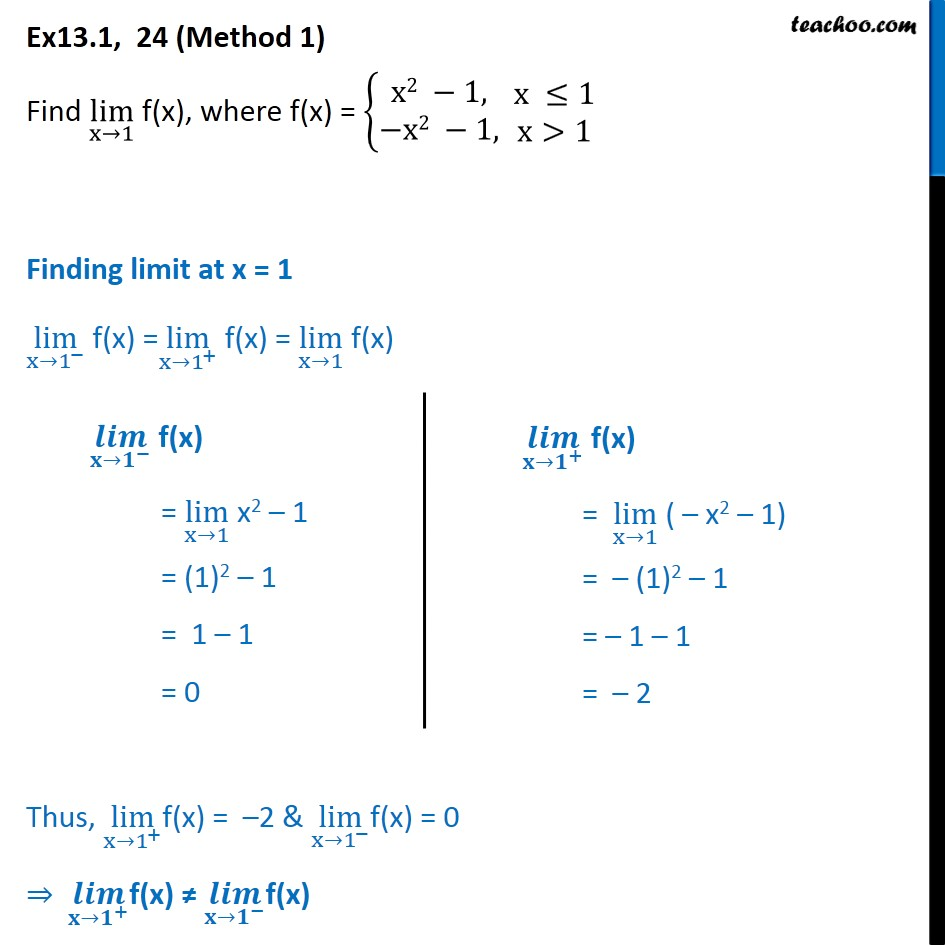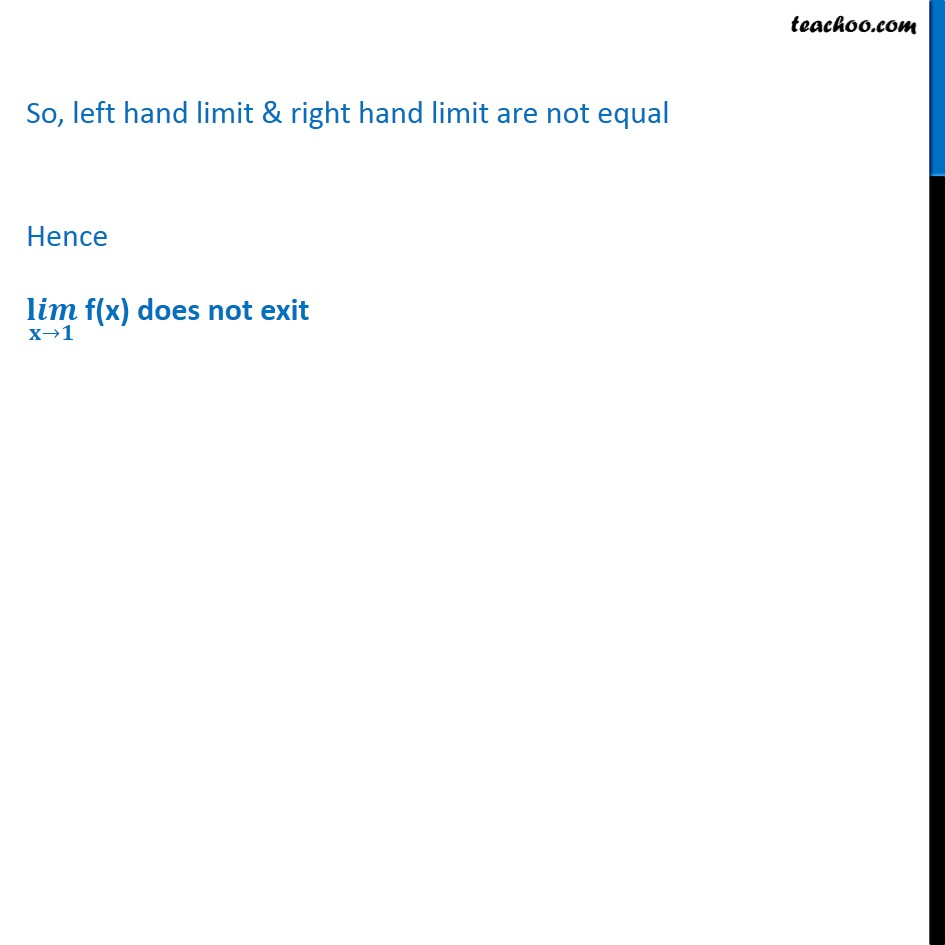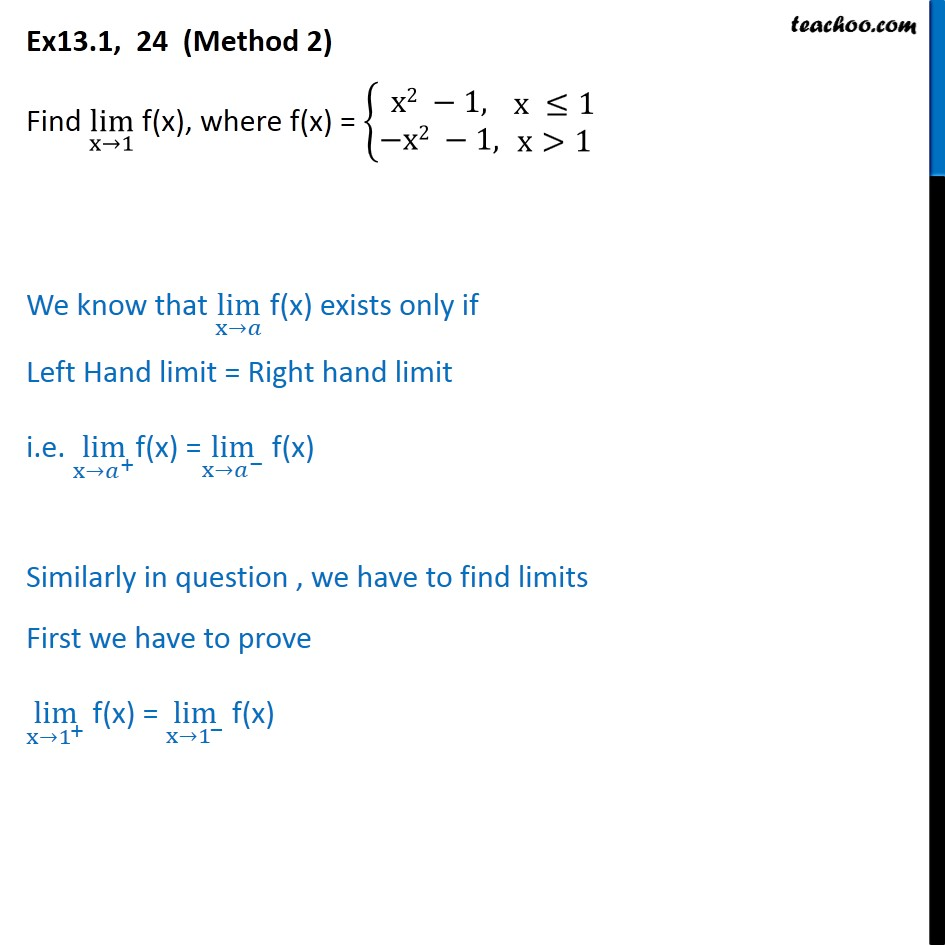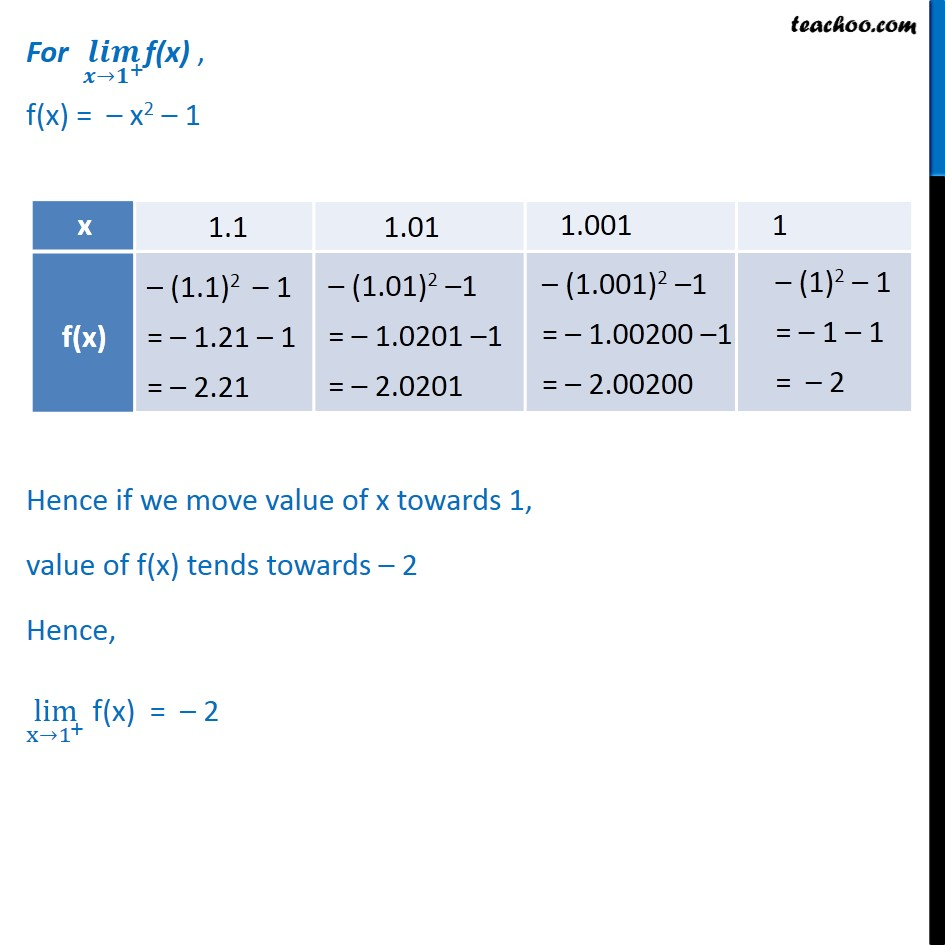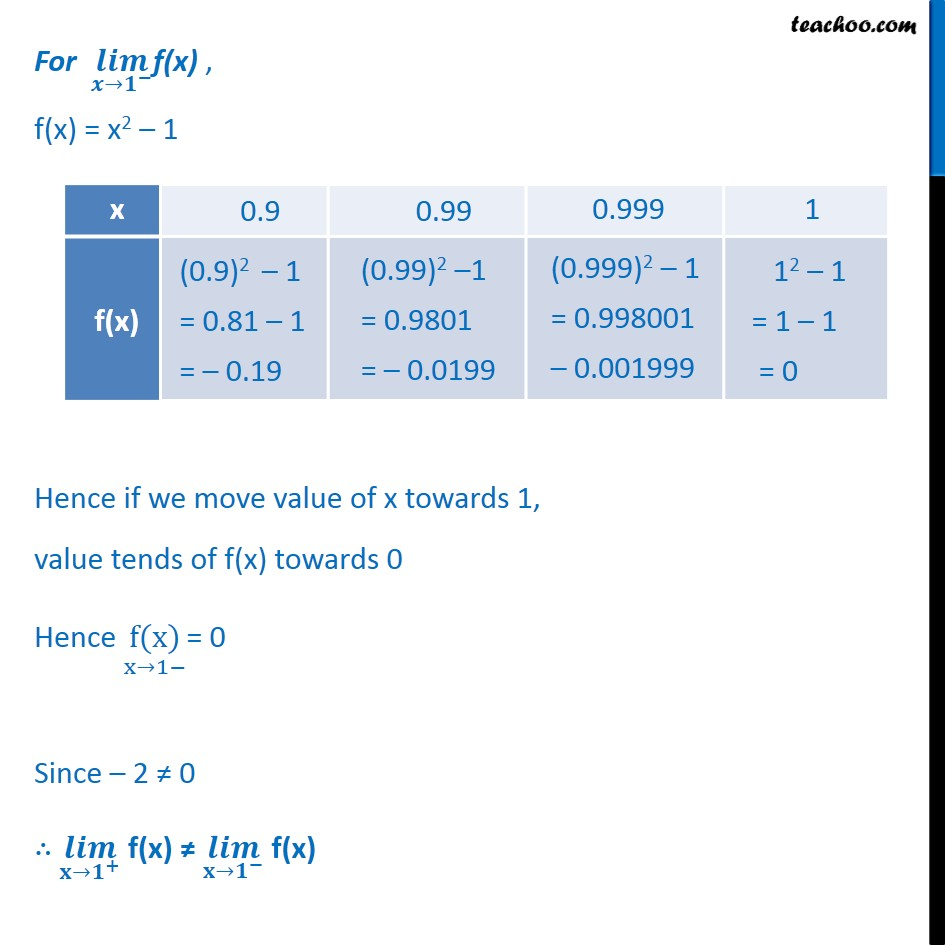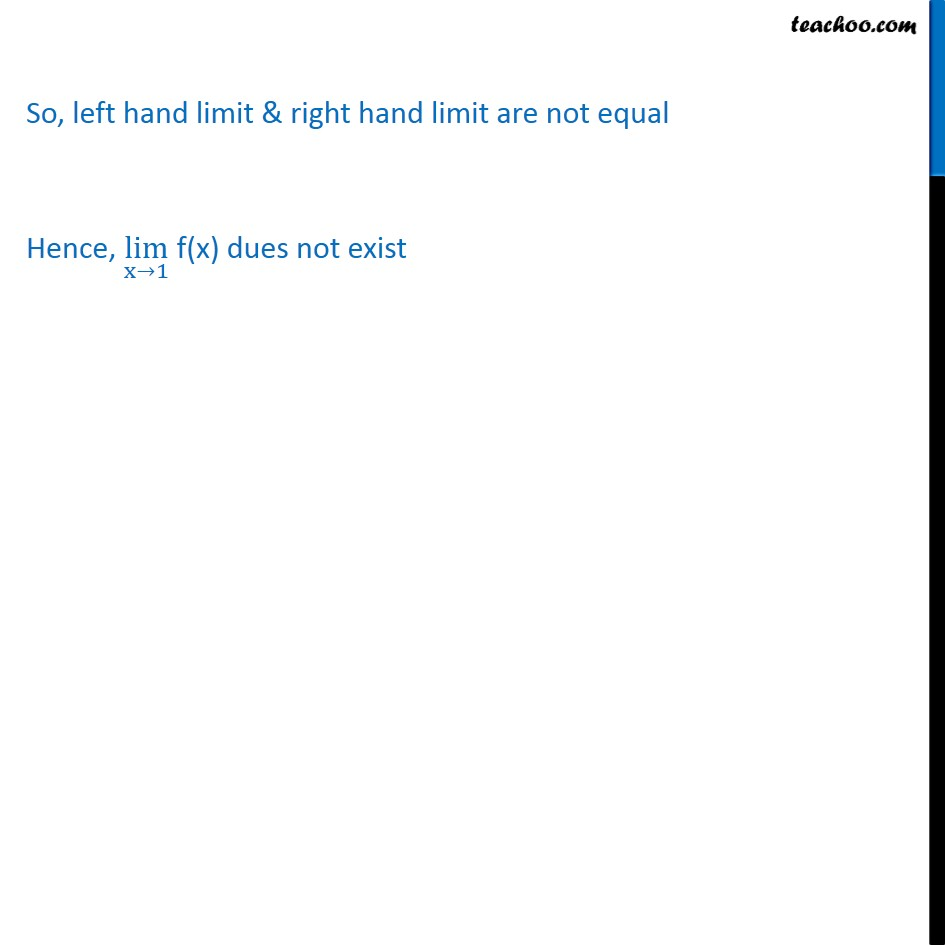1. Chapter 13 Class 11 Limits and Derivatives
2. Serial order wise
3. Ex 13.1

Transcript

Ex 13.1, 24 (Method 1) Find lim﷮x→1﷯ f(x), where f(x) = x2 −1,﷮−x2 −1,﷯﷯ x ≤1﷮x>1﷯ Finding limit at x = 1 lim﷮ x→1﷮−﷯﷯ f(x) = lim﷮ x→1﷮+﷯﷯ f(x) = lim﷮x→1﷯ f(x) Thus, lim﷮ x→1﷮+﷯﷯f(x) = –2 & lim﷮ x→1﷮−﷯﷯f(x) = 0 ⇒ 𝒍𝒊𝒎﷮ 𝐱→𝟏﷮+﷯﷯f(x) ≠ 𝒍𝒊𝒎﷮ 𝐱→𝟏﷮−﷯﷯f(x) So, left hand limit & right hand limit are not equal Hence 𝐥𝒊𝒎﷮𝐱→𝟏﷯ f(x) does not exit Ex13.1, 24 (Method 2) Find lim﷮x→1﷯ f(x), where f(x) = x2 −1,﷮−x2 −1,﷯﷯ x ≤1﷮x>1﷯ We know that lim﷮x→𝑎﷯ f(x) exists only if Left Hand limit = Right hand limit i.e. lim﷮ x→𝑎﷮+﷯﷯f(x) = lim﷮ x→𝑎﷮−﷯﷯ f(x) Similarly in question , we have to find limits First we have to prove lim﷮x→ 1﷮+﷯﷯ f(x) = lim﷮x→ 1﷮−﷯﷯ f(x) For 𝒍𝒊𝒎﷮ 𝒙→𝟏﷮+﷯﷯f(x) , f(x) = – x2 – 1 Hence if we move value of x towards 1, value of f(x) tends towards – 2 Hence, lim﷮x→ 1﷮+﷯﷯ f(x) = – 2 For 𝒍𝒊𝒎﷮ 𝒙→𝟏﷮−﷯﷯f(x) , f(x) = x2 – 1 Hence if we move value of x towards 1, value tends of f(x) towards 0 Hence f(x)﷮x→1−﷯= 0 Since – 2 ≠ 0 ∴ 𝒍𝒊𝒎﷮𝐱→ 𝟏﷮+﷯﷯ f(x) ≠ 𝒍𝒊𝒎﷮𝐱→ 𝟏﷮−﷯﷯ f(x) So, left hand limit & right hand limit are not equal Hence, lim﷮x→1﷯ f(x) dues not exist

Ex 13.1